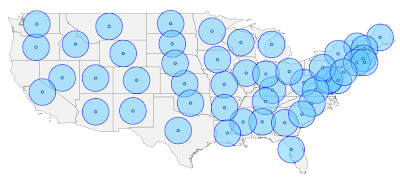# GeoScript

## Monday, May 10, 2010

### Calculating Centroids with JavaScriptIn a previous post, Jared described how to use the Groovy GeoScript to create a new Shapefile based on centroids of features in an existing Shapefile. Today, I'll do the same using the JavaScript implementation.

One significant difference from the other implementations is that there is only one layer type in the JavaScript flavor of GeoScript. To create a layer representing a Shapefile, I'll create a directory workspace and get the layer from that.

In this example, I'll also use the `clone` methods to create a new layer schema and new features. These `clone` methods allow for new objects to be created with modified properties. This is useful in creating the schema for the centroids layer, because we want the same fields as the states layer with the exception of the geometry field. In this case, we're creating a layer with point geometries instead of multi-polygon geometries.

Without further ado, here is the code:
`// import Directory and Layer constructorsvar Directory = require("geoscript/workspace").Directory;var Layer = require("geoscript/layer").Layer;// create a directory workspace from an existing directory on diskvar dir = new Directory("data/shapefiles");// create a layer based on an existing shapefile in the directoryvar states = dir.get("states");// create a new schema with a Point geometry type instead of MultiPolygonvar schema = states.schema.clone({    // give the schema a new name    name: "centroids",     fields: [        // overwrite existing field named "the_geom"        {name: "the_geom", type: "Point", projection: "EPSG:4326"}    ]});// create a new temporary layer with the new schemavar centroids = new Layer({    schema: schema});// add the layer to existing workspace (this creates a new shapefile on disk)dir.add(centroids);// iterate through the state features to create features with state centroidsstates.features.forEach(function(state) {    var centroid = state.clone({        schema: schema,        values: {            // overwrite the geometry value with the state centroid            the_geom: state.geometry.centroid        }    });    // add the new feature to the layer (this writes to the shapefile)    centroids.add(centroid);});`

The GeoScript `viewer` module exports a `draw` method. You can use this to render a layer, or arrays of geometies or features.

`// use the viewer module to draw collections of features or geometriesvar draw = require("geoscript/viewer").draw;var geometries = [];// add all state geometries to the arraystates.features.forEach(function(state) {    geometries.push(state.geometry);});// add buffered centroids so they will be visiblecentroids.features.forEach(function(centroid) {    geometries.push(centroid.geometry.buffer(0.5));});// draw all geometriesdraw(geometries);`

You'll find this example in the examples directory of the repository. See the JavaScript API documentation for more detail on using GeoScript.

## Friday, May 7, 2010

### Buffering Features with Groovy

In my last post, I calculated centroids from one shapefile and saved them to another using a GeoScript Groovy script. This time I buffer these centroids and save them to a polygon shapefile. Since GeoScript is based on the Java Topology Suite library you can take advantage of any of its geometry operations - intersection, union and difference.
`// Import Geoscript modulesimport geoscript.layer.*import geoscript.feature.*import geoscript.geom.*// Get the Shapefile we want to bufferShapefile shp = new Shapefile('states_centroids.shp')// Create a new Schema  but with Polygon GeometrySchema schema = shp.schema.changeGeometryType('Polygon','states_buffers')// Create the new LayerLayer bufferLayer = shp.workspace.create(schema)// Specify the buffer distance double distance = 2 // decimal degrees// Iterate through each Feature using a closureshp.features.each{f ->  // Create a Map for the new attributes  Map attributes = [:]  // Set attribute values  f.attributes.each{k,v ->      if (v instanceof Geometry) {          attributes[k] = v.buffer(distance)      }      else {          attributes[k] = v      }  }  // Create a new Feature with the new attributes  Feature feature = schema.feature(attributes, f.id)  // Add it to the buffer Layer  bufferLayer.add(feature)}`

The Layer.getCursor() method, used in the last post, reads one Feature at a time. In this post I use Layer.getFeatures(). This method reads all of the Features in to a list. Here is what you get:## Introducing GeoScript

GeoScript adds geo capabilities to dynamic scripting languages such as JavaScript, Python, Scala and Groovy.